### Test of Hypotheses

Consider a general linear hypothesis of the form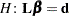, whereis a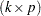matrix. It is assumed that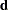is such that this hypothesis is linearly consistent—that is, that there exists somefor which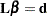. This is always the case ifis in the column space of, ifhas full row rank, or if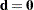; the latter is the most common case. Since many linear models have a rank-deficientmatrix, the question arises whether the hypothesis is testable. The idea of testability of a hypothesis is—not surprisingly—connected to the concept of estimability as introduced previously. The hypothesisis testable if it consists of estimable functions.

There are two important approaches to testing hypotheses in statistical applications—the reduction principle and the linear inference approach. The reduction principle states that the validity of the hypothesis can be inferred by comparing a suitably chosen summary statistic between the model at hand and a reduced model in which the constraintis imposed. The linear inference approach relies on the fact that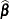is an estimator ofand its stochastic properties are known, at least approximately. A test statistic can then be formed using, and its behavior under the restrictioncan be ascertained.

The two principles lead to identical results in certain—for example, least squares estimation in the classical linear model. In more complex situations the two approaches lead to similar but not identical results. This is the case, for example, when weights or unequal variances are involved, or whenis a nonlinear estimator.

#### Reduction Tests

The two main reduction principles are the sum of squares reduction test and the likelihood ratio test. The test statistic in the former is proportional to the difference of the residual sum of squares between the reduced model and the full model. The test statistic in the likelihood ratio test is proportional to the difference of the log likelihoods between the full and reduced models. To fix these ideas, suppose that you are fitting the model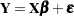, where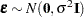. Suppose that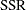denotes the residual sum of squares in this model and that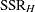is the residual sum of squares in the model for whichholds. Then under the hypothesis the ratio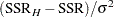follows a chi-square distribution with degrees of freedom equal to the rank of. Maybe surprisingly, the residual sum of squares in the full model is distributed independently of this quantity, so that under the hypothesis,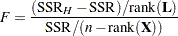follows andistribution with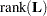numerator and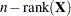denominator degrees of freedom. Note that the quantity in the denominator of thestatistic is a particular estimator of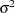—namely, the unbiased moment-based estimator that is customarily associated with least squares estimation. It is also the restricted maximum likelihood estimator ofif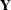is normally distributed.

In the case of the likelihood ratio test, suppose that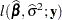denotes the log likelihood evaluated at the ML estimators. Also suppose that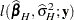denotes the log likelihood in the model for whichholds. Then under the hypothesis the statistic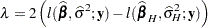follows approximately a chi-square distribution with degrees of freedom equal to the rank of. In the case of a normally distributed response, the log-likelihood function can be profiled with respect to. The resulting profile log likelihood is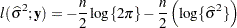and the likelihood ratio test statistic becomes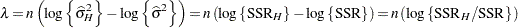The preceding expressions show that, in the case of normally distributed data, both reduction principles lead to simple functions of the residual sums of squares in two models. As Pawitan (2001, p. 151) puts it, there is, however, an important difference not in the computations but in the statistical content. The least squares principle, where sum of squares reduction tests are widely used, does not require a distributional specification. Assumptions about the distribution of the data are added to provide a framework for confirmatory inferences, such as the testing of hypotheses. This framework stems directly from the assumption about the data’s distribution, or from the sampling distribution of the least squares estimators. The likelihood principle, on the other hand, requires a distributional specification at the outset. Inference about the parameters is implicit in the model; it is the result of further computations following the estimation of the parameters. In the least squares framework, inference about the parameters is the result of further assumptions.

#### Linear Inference

The principle of linear inference is to formulate a test statistic forthat builds on the linearity of the hypothesis about. For many models that have linear components, the estimator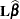is also linear in. It is then simple to establish the distributional properties ofbased on the distributional assumptions aboutor based on large-sample arguments. For example,might be a nonlinear estimator, but it is known to asymptotically follow a normal distribution; this is the case in many nonlinear and generalized linear models.

If the sampling distribution or the asymptotic distribution ofis normal, then one can easily derive quadratic forms with known distributional properties. For example, if the random vector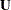is distributed as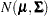, then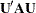follows a chi-square distribution with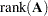degrees of freedom and noncentrality parameter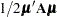, provided that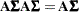.

In the classical linear model, suppose thatis deficient in rank and that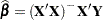is a solution to the normal equations. Then, if the errors are normally distributed,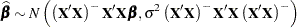Becauseis testable,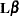is estimable, and thus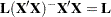, as established in the previous section. Hence,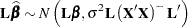The conditions for a chi-square distribution of the quadratic form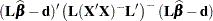are thus met, provided that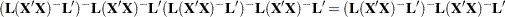This condition is obviously met if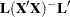is of full rank. The condition is also met if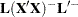is a reflexive inverse (a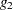-inverse) of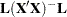.

The test statistic to test the linear hypothesisis thus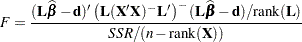and it follows andistribution withnumerator and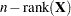denominator degrees of freedom under the hypothesis.

This test statistic looks very similar to thestatistic for the sum of squares reduction test. This is no accident. If the model is linear and parameters are estimated by ordinary least squares, then you can show that the quadratic form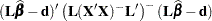equals the differences in the residual sum of squares,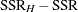, whereis obtained as the residual sum of squares from OLS estimation in a model that satisfies. However, this correspondence between the two test formulations does not apply when a different estimation principle is used. For example, assume that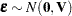and thatis estimated by generalized least squares: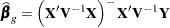The construction ofmatrices associated with hypotheses in SAS/STAT software is frequently based on the properties of thematrix, not of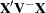. In other words, the construction of thematrix is governed only by the design. A sum of squares reduction test for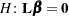that uses the generalized residual sum of squares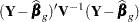is not identical to a linear hypothesis test with the statistic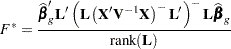Furthermore,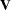is usually unknown and must be estimated as well. The estimate fordepends on the model, and imposing a constraint on the model would change the estimate. The asymptotic distribution of the statistic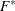is a chi-square distribution. However, in practical applications thedistribution withnumerator and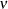denominator degrees of freedom is often used because it provides a better approximation to the sampling distribution ofin finite samples. The computation of the denominator degrees of freedom, however, is a matter of considerable discussion. A number of methods have been proposed and are implemented in various forms in SAS/STAT (see, for example, the degrees-of-freedom methods in the MIXED and GLIMMIX procedures).